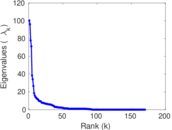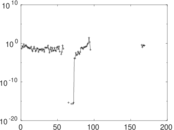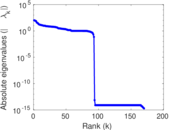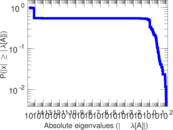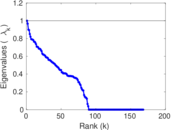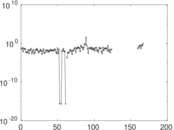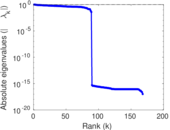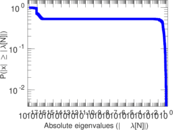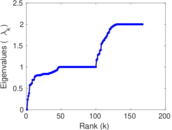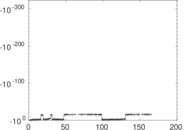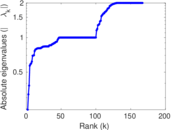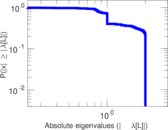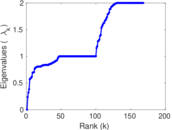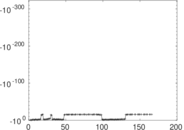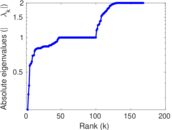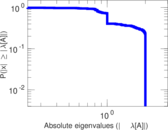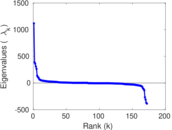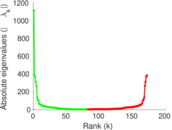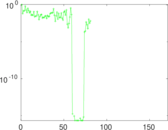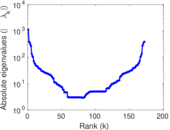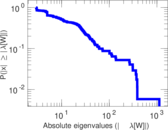# Wikiquote edits (pa)

This is the bipartite edit network of the Punjabi Wikisource. It contains users and pages from the Punjabi Wikisource, connected by edit events. Each edge represents an edit. The dataset includes the timestamp of each edit.

 Code `qpa` Internal name `edit-pawikisource` Name Wikiquote edits (pa) Data source http://dumps.wikimedia.org/ AvailabilityDataset is available for download Consistency checkDataset passed all tests Category Authorship network Dataset timestamp 2017-10-20 Node meaning User, article Edge meaning Edit Network formatBipartite, undirected Edge typeUnweighted, multiple edges Temporal dataEdges are annotated with timestamps

## Statistics

 Size n = 1,113 Left size n1 = 172 Right size n2 = 941 Volume m = 5,660 Unique edge count m̿ = 3,144 Wedge count s = 634,993 Claw count z = 136,046,311 Cross count x = 26,063,786,611 Square count q = 310,509 4-Tour count T4 = 5,036,244 Maximum degree dmax = 1,076 Maximum left degree d1max = 1,076 Maximum right degree d2max = 276 Average degree d = 10.170 7 Average left degree d1 = 32.907 0 Average right degree d2 = 6.014 88 Fill p = 0.019 425 2 Average edge multiplicity m̃ = 1.800 25 Size of LCC N = 1,100 Diameter δ = 7 50-Percentile effective diameter δ0.5 = 1.853 01 90-Percentile effective diameter δ0.9 = 3.490 71 Median distance δM = 2 Mean distance δm = 2.607 87 Gini coefficient G = 0.659 823 Balanced inequality ratio P = 0.251 767 Left balanced inequality ratio P1 = 0.102 120 Right balanced inequality ratio P2 = 0.361 484 Relative edge distribution entropy Her = 0.773 208 Power law exponent γ = 1.963 03 Tail power law exponent γt = 1.721 00 Tail power law exponent with p γ3 = 1.721 00 p-value p = 0.778 000 Left tail power law exponent with p γ3,1 = 1.551 00 Left p-value p1 = 0.736 000 Right tail power law exponent with p γ3,2 = 7.881 00 Right p-value p2 = 0.000 00 Degree assortativity ρ = −0.219 835 Degree assortativity p-value pρ = 1.028 76 × 10−35 Spectral norm α = 100.279 Algebraic connectivity a = 0.236 030 Spectral separation |λ1[A] / λ2[A]| = 1.044 26 Controllability C = 926 Relative controllability Cr = 0.832 734

## Plots

### Fruchterman–Reingold graph drawing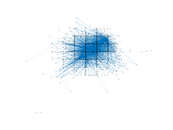### Degree distribution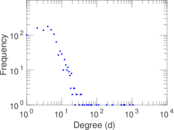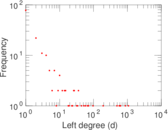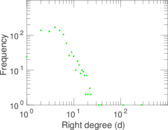### Cumulative degree distribution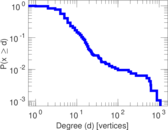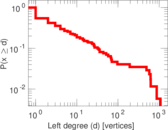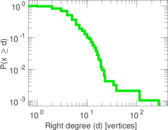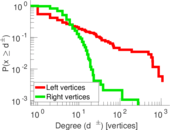### Lorenz curve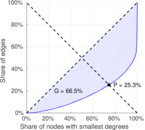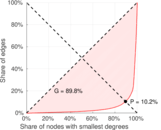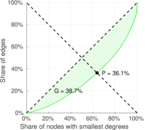### Spectral distribution of the adjacency matrix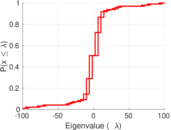### Spectral distribution of the normalized adjacency matrix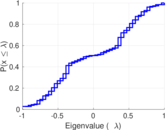### Spectral distribution of the Laplacian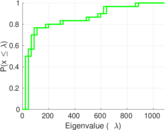### Spectral graph drawing based on the adjacency matrix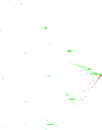### Spectral graph drawing based on the Laplacian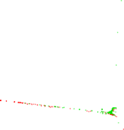### Spectral graph drawing based on the normalized adjacency matrix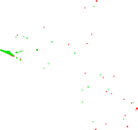### Degree assortativity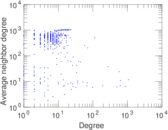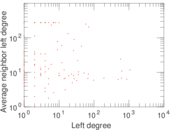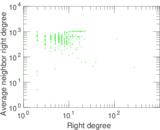### Zipf plot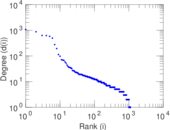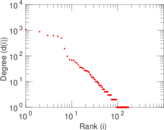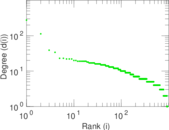### Hop distribution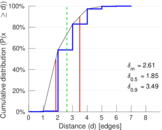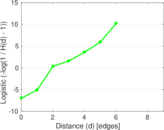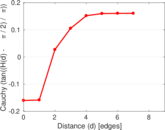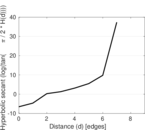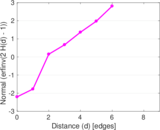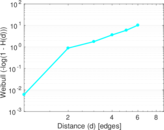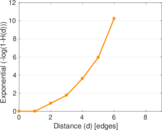### Double Laplacian graph drawing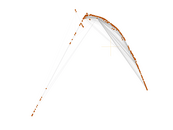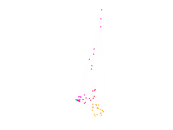### Delaunay graph drawing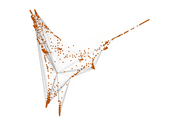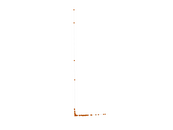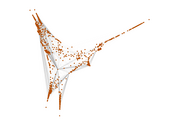### Edge weight/multiplicity distribution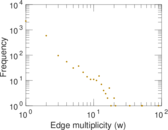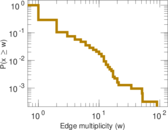### Temporal distribution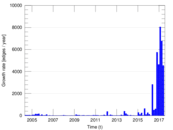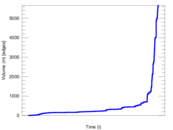### Temporal hop distribution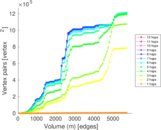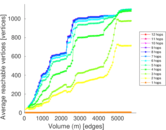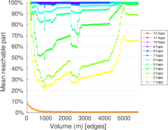### Diameter/density evolution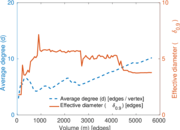### Matrix decompositions plots